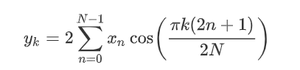Open in App
Not now

# Python – scipy.fft.dct() method

• Difficulty Level : Hard
• Last Updated : 01 Oct, 2020

With the help of scipy.fft.dct() method, we can compute the discrete cosine transform by selecting different types of sequences and return the transformed array by using this method.Syntax :

```scipy.fft.dct(x, type=2)
```

Return value: It will return the transformed array.

Example #1: In this example, we can see that by using scipy.fft.dct() method, we are able to get the discrete cosine transform by selecting different types of sequences by default it’s 2.

## Python3

 `# import scipy ` `from` `scipy ``import` `fft ` ` `  `# Using scipy.fft.dct() method ` `gfg ``=` `fft.dct([``1``, ``2``, ``3``, ``4``]) ` ` `  `print``(gfg)`

Output :

```[20.00000000  -6.30864406  0.00000000  -0.44834153]
```

Example #2 :

## Python3

 `# import scipy ` `from` `scipy ``import` `fft ` ` `  `# Using scipy.fft.dct() method ` `gfg ``=` `fft.dct([``-``6``, ``5``, ``-``4``, ``3``, ``-``2``, ``1``], ``3``) ` ` `  `print``(gfg)`

Output :

```[ -0.50866619  -0.58578644  -0.79439535  -1.34919819  -3.41421356
-29.34774027]
```
My Personal Notes arrow_drop_up
Related Articles